lang="en-GB"> Towards understanding IMU: Frames of reference used to represent IMU orientation and how to visualize the circuit orientation using Vpython library - Atadiat

# Towards understanding IMU: Frames of reference used to represent IMU orientation and how to visualize the circuit orientation using Vpython library

We explained how the accelerometer and gyroscope work in the IMU in the previous part. We showed the characteristics of each one and derived the equations needed to calculate the pitch, roll and yaw angles. In addition, we presented how to enhance extracted information immunity to noise for each. In another article, we presented the magnetometer with the needed calibration process and how to extract the azimuth information from it. In this part, we will discuss the most common frames of reference used with IMUs, Inertial and body frames, and how to convert between them. We will discuss the limitation of representing the system orientation with the pitch, roll and yaw angles at 90 degrees, which are used in the Euler formula. This limitation is presented with Gimbal lock as we are going to see. Then we are presenting the alternative axis-angle and Quaternions formulations for orientation. Finally, we will show how to use the Vpython library to visualize the circuit orientation in a 3D environment.

IMU series:

Check a capstone project that utilizes the concepts found in the 3 past parts of this series. Qibla compass with tilt compensation.

## Frames of reference used with IMU data

The values of any point in 3D world are relative to a specific reference frame (coordinate axes). One of the most common frames used in the IMU sensor is the inertial frame, which is fixed on the earth’s surface, and the body frame, which is aligned with the sensor’s body. Intermediate frames are needed when converting from the inertial frame to the body frame. These intermediate frames are vehicle-1 and vehicle-2. Inertial and body frames are not the only frames of reference in literature, but it is sufficient to mention these two common frames.

The inertial frame is fixed and has an x-axis towards the north direction, a y-axis towards the east, and a z-axis toward the earth’s gravity.An image showing the inertial frame, with the x-axis towards the north, the y-axis towards the east, and the z-axis towards the gravity direction. Image source: CH Robotics’ application note AN-1005

Suppose that the plane is displaced from the north by the angle of yaw. Yaw angle will affect the x and y axes, as in the figure below, and this form the intermediate frame vehicle-1.The vehicle-1 frame resulting from adding the Yaw angle to the inertial frame. This affects the x and y axes only. Image source: CH Robotics’ application note AN-1005

To convert a vector from the inertial frame to the vehicle-1 frame, we use a rotation matrix. By multiplying the rotation matrix with the vector values in the inertial frame we get the values of the same vector but relative to the new frame.

The rotation matrix can be derived using the trigonometric laws and is given in the illustration below:The derivation of the rotation matrix between the internal frame and vehicle-1 frame using a point P as an example. Assuming the rotation of the axes with a yaw angle.

The matrix shown in the previous figure can be generalized over a three-dimensional space, as the Yaw angle does not cause a change in the z-axis, and thus the generalized matrix is: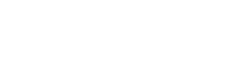The vehicle-2 frame results from adding a Pitch angle for the vehicle-1 frame and the rotation matrix is characterized this time as being fixed at the y-axis because the pitch is by rotating across the y-axis.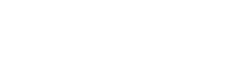The vehicle-2 frame resulting from adding pitch angle to the vehicle-1 frame. This affects the x and z axes only. Image source: CH Robotics’ application note AN-1005

To reach the body frame, we add the roll angle that is around the X axis, so this time the rotation matrix is fixed in the X axis.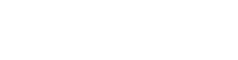The rotation matrix from inertial frame to body frame can be expressed in the following way: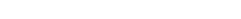## Is a representation of orientation with the three angles enough?

The Euler angles is one system used to express the orientation of a body.  Euler angles fail when the pitch angle approaches 90 degrees. This phenomenon is known as Gimbal Lock and can be mathematically proven, but we will not go into its details this time. It is sufficient to know that this phenomenon means the loss of one of the three degrees of freedom of the system at the angle of 90 degrees. References can be found for a full explanation of this phenomenon. Because of this limitation, the Quaternion representation is used, which we will explain later. Quaternion representation does not suffer from the gimbal lock phenomenon.

It should be noted that the movement of the body in Euler’s representation takes place through a series of movements across the three axes (tilt – roll – yaw), and this is of course from a mathematical point of view as we actually move the body absolutely freely in reality. However, the mathematical model from which we derived the equations was based on a series of sequential movements.An example of a series of movements needed to move the arrow from the initial state (number one) to the target state (number four). We consider overlapping gears the 1st gear movement  causes the 2nd and 3rd level gears to move, the 2nd level gear movement will cause the 3rd level gear to move. The primary gear is in green, the second gear is in blue, and the final gear is in red. Images used are from the YouTube video Euler (gimbal lock) Explained from GuerrillaCG channel. Although we do not actually follow these steps when we want to move the body, but some mathematical models, including Euler’s formula, assume the existence of these overlapping gears, and this is why the gimbal lock phenomenon occurs.

In order to get rid of the gimbal lock phenomenon, we rely on a model in which the movement takes place in one go, assuming a vector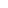and an angle of rotation around this ray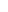.

The Axis-angle representation is an alternative representation of Euler angles. This alternative representation uses a vector v in addition to the anglerepresenting the angle of the body around this vector. We can convert from Axis-angle to quaternion as we will explain in the next paragraph.Representing the body heading with a vector v, and rotation angle /theta. This representation is called Axis-angle representation. The picture is taken from lecture slides of ee267 course from Stanford University

## Quaternion Representation

The quaternion is an alternative representation of Euler’s one. It is possible to switch between the two representation systems using specific mathematical formulas that we will mention later. The quaternion is a vector of four numbers, 3 of which are imaginary parts and one is a real number that expresses the vector length. Quaternion is an extension of the complex numbers.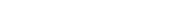To convert from axis-angle to quaternion, we use the following formula. Please note that axis-angle is explained in the previous figure:To convert a vector from the inertial frame to the body frame, we use the following equation: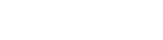We notice that, we added a zero to the axis-angle representation in the conversion equation. We need to know that the multiplication between a quaternion 1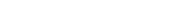and a quaternion 2is :The conjugate of a quaternion is the inverse of the magical part only of the quaternion: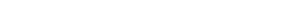We notice that we need to do two multiplications for converting between the two frames of reference, but we can use and build a rotation matrix directly, which is: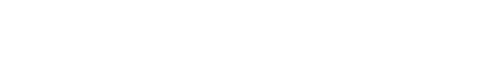And it would finally be: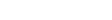To get the pitch, roll and yaw angles we use the following:We will use quaternions practically next time.

## Visualization using Vpython

We will use the Vpython library to visualize the circuit and represent ist orientation in 3D. Let’s initially represent the circuit as a vector with components of x, y, z. To calculate these three values, we start from the three angles calculated through the sensor, which are the angles of pitch, roll, and yaw. The Yaw angle is formed between the x-axis and the projection of the vector on the XY surface. The amplitude of this projection is h. Using the laws of trigonometry, we find that: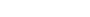We assume that h length is 1.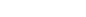The third component is z: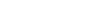In overall: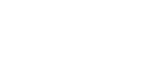An illustration of how to calculate the components of the vector representing the body
The coordinates axis in the VPython library are shown below. We note that the y-axis replaces the z-axis in the representation Vpython adopted, and because of that, the calculations of the previous figure remain correct, but we reverse between the values of z and y .A figure showing the frame used in the VPython library. The figure shows that the length of the body is on the x-axis, its height is on the y-axis, and its width is on the z-axis. There are also two main vectors for the body, which is the axis vector and the up vector.

The reference code used to visualize the circuit orientation is a code found in Paul McWhorter’s series of lessons about IMU sensor, specifically lesson 18. A modification has been made which is setting an image to the 3D box. The original code relies on drawing approximate three-dimensional shapes to express the circuit. The Vpython library does not allow setting an image for the body on top or bottom, only on the sides. To solve this problem, I set the image on the right side and rotated the body by 90 degrees. This explains the following two lines:

bBoard=box(texture={'file':'board.jpg','place':['right'] },length=.2,width=2,height=6,opacity=.8)
bBoard.rotate(angle=np.pi/2,axis=vector(0,0,1))


Please refer to the previous figure for the Vpython axes order and dimensions in Vpython environment. We will assign three fixed axes in the figure that express the axes of the environment in colour (red: x, green: y, blue: z):

xarrow=arrow(lenght=2, shaftwidth=.1, color=color.red,axis=vector(1,0,0))
yarrow=arrow(lenght=2, shaftwidth=.1, color=color.green,axis=vector(0,1,0))
zarrow=arrow(lenght=4, shaftwidth=.1, color=color.blue,axis=vector(0,0,1))



We will add three more axes aligned to the circuit body. We will handle moving them later in the program

frontArrow=arrow(length=4,shaftwidth=.1,color=color.purple,axis=vector(1,0,0))
upArrow=arrow(length=1,shaftwidth=.1,color=color.magenta,axis=vector(0,1,0))
sideArrow=arrow(length=2,shaftwidth=.1,color=color.orange,axis=vector(0,0,1))



We extract the three angles values from the received data through serial communication by sending the three angles in degrees and separated by comma, then we calculate the vector components:

	body_x,body_y,body_z = cos(yaw)*cos(pitch),sin(pitch),sin(yaw)*cos(pitch)


We call this vector an axis vector as the library calls it

	k=vector(body_x,body_y,body_z)


We need to find the orthogonal axes aligned with body which move according to the movement of the body. This is done mathematically through the cross product of the k vector with a temporary intermediate vector, which is a vector on the y-axis.

	k=vector(body_x,body_y,body_z)
y=vector(0,1,0)
s=cross(k,y)
v=cross(s,k)


We should note that the derived equations for calculating the x, y, z components will not change if the rolling angle changes as only pitch and roll angles are used. We will use the Rodrigues rotation formula, to calculate the new vector components after rolling.

Rodrigues rotation formula states that if v is a vector and k is a unit vector describing an axis of rotation about which v rotates by an angle θ according to the right-hand rule. To map this to the Vpython environment, the v is the up vector, k is the axis vector, and the angle θ is actually the rolling vector which is indeed around the k vector. Rodrigues formula for the rotated vector vrot is: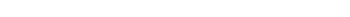Noting that the vectors v and k are perpendicular, and therefore their inner product is equal to zero. Therefore, we delete the last component of the Rodrigos formula. By deleting the last component, it becomes: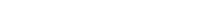This is what we find in the python code:

vrot=v*cos(roll)+cross(k,v)*sin(roll)


We assign the box’s up and axis vectors as follows. Please note that we have rotated the box by 90 degrees initially so we set the up vector to k and axis vector to vrot :

	bBoard.axis=vrot
bBoard.up=k


We assign the orthogonal axes aligned with the body which move according to the movement of the body.

	frontArrow.axis=k
sideArrow.axis=cross(k,vrot)
upArrow.axis=v


Before running the code remember to change the port name depending on your machine:

from vpython import *
from time import *
from visual import *
import numpy as np
import math
import serial

sleep(1)

canvas(title='IMU Data 3D Visualization', caption='A caption')

scene.range=5
scene.forward=vector(-1,-1,-1)

scene.width=800
scene.height=800

xarrow=arrow(lenght=2, shaftwidth=.1, color=color.red,axis=vector(1,0,0))
yarrow=arrow(lenght=2, shaftwidth=.1, color=color.green,axis=vector(0,1,0))
zarrow=arrow(lenght=4, shaftwidth=.1, color=color.blue,axis=vector(0,0,1))

frontArrow=arrow(length=4,shaftwidth=.1,color=color.purple,axis=vector(1,0,0))
upArrow=arrow(length=1,shaftwidth=.1,color=color.magenta,axis=vector(0,1,0))
sideArrow=arrow(length=2,shaftwidth=.1,color=color.orange,axis=vector(0,0,1))

bBoard=box(texture={'file':'board.jpg','place':['right'] },length=.2,width=2,height=6,opacity=.8)
bBoard.rotate(angle=np.pi/2,axis=vector(0,0,1))

while (True):
pass
roll,pitch,yaw= .0,.0,.0
try:
dataPacket=str(dataPacket,'utf-8')
splitPacket=dataPacket.split(",")
yaw = -yaw
except:
pass
log = 'Roll={},Pitch={},Yaw={}'.format(roll*toDeg, pitch*toDeg,yaw*toDeg)
print(log)
scene.caption = log

rate(50)

body_x,body_y,body_z = cos(yaw)*cos(pitch),sin(pitch),sin(yaw)*cos(pitch)
k=vector(body_x,body_y,body_z)

y=vector(0,1,0)
s=cross(k,y)
v=cross(s,k)
vrot=v*cos(roll)+cross(k,v)*sin(roll)

frontArrow.axis=k
sideArrow.axis=cross(k,vrot)
upArrow.axis=v
bBoard.axis=vrot
bBoard.up=k
sideArrow.length=2
frontArrow.length=4
upArrow.length=1
bBoard.width = 2
bBoard.height = 6
bBoard.length = .2